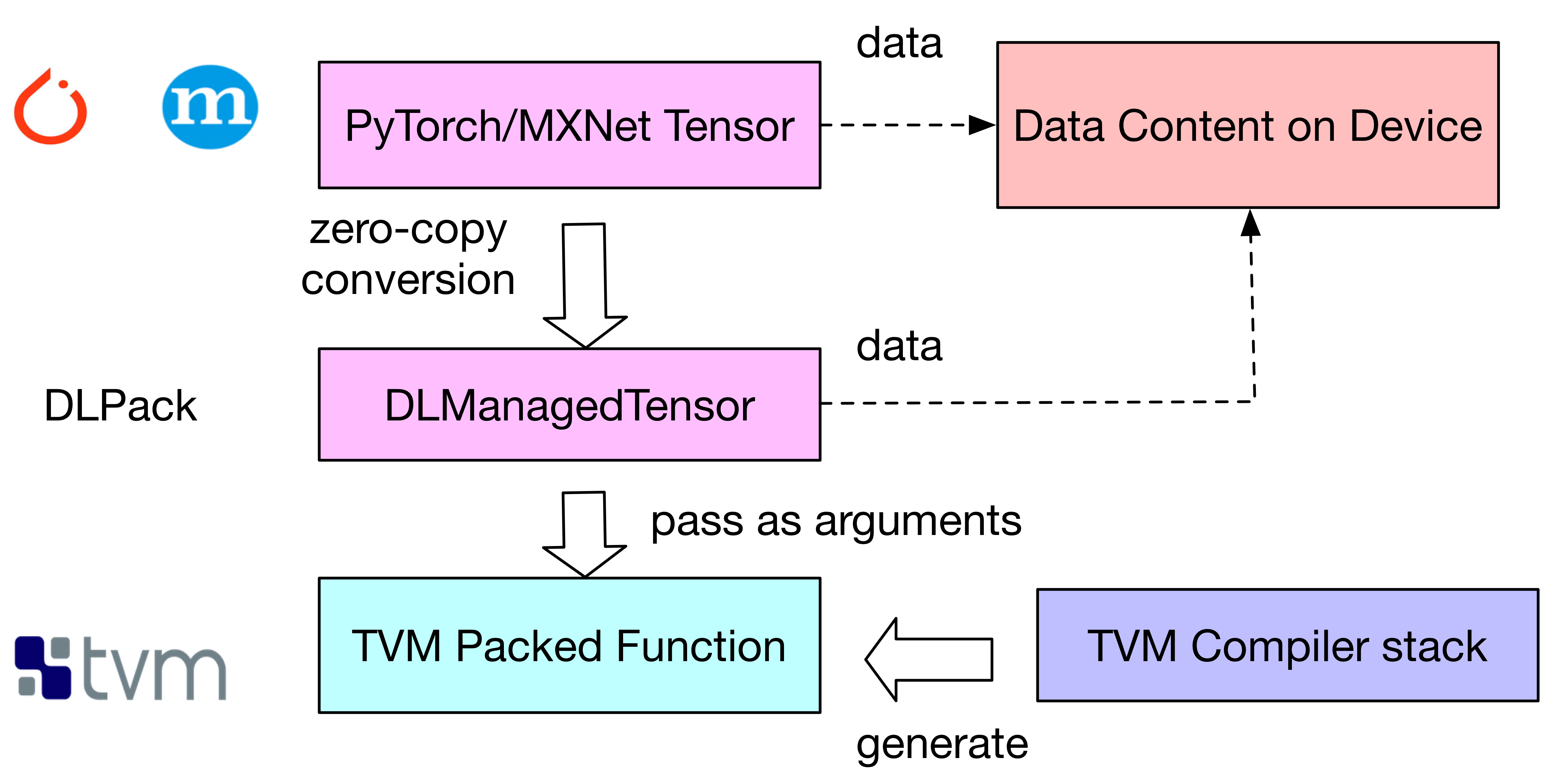# Building a Cross-Framework Deep Learning Compiler via DLPack

Deep learning frameworks such as Tensorflow, PyTorch, and ApacheMxNet provide a powerful toolbox for quickly prototyping and deploying deep learning models. Unfortunately, their ease-of-use has often come at the cost of fragmentation: it is only easy to use each framework in isolation. Vertical integration has made development streamlined for common use cases, but venturing off of the beaten path can be tricky.

One scenario that is poorly supported is passing tensors directly from one framework to another in memory, without any data duplication or copies. Supporting such a use case would enable users to string together pipelines where certain operators are better supported in one framework (or faster) than another efficiently. A shared data representation between frameworks would also bridge this gap, and allow compiler stacks to target a single format when generating code for operators.

DLPack is an intermediate in-memory representation standard for tensor data structures. With DLPack as a common representation, we can leverage TVM in scripts written for frameworks that traditionally could only rely on vendor-provided libraries. TVM packed functions can operate on DLPack tensors, providing wrappers bridging tensor data structures from frameworks such as PyTorch and MxNet with zero-data-copy.

DLPack presents a simple, portable in-memory data structure:

``````/*!
* \brief Plain C Tensor object, does not manage memory.
*/
typedef struct {
/*!
* \brief The opaque data pointer points to the allocated data.
*  This will be CUDA device pointer or cl_mem handle in OpenCL.
*  This pointer is always aligns to 256 bytes as in CUDA.
*/
void* data;
/*! \brief The device context of the tensor */
DLContext ctx;
/*! \brief Number of dimensions */
int ndim;
/*! \brief The data type of the pointer*/
DLDataType dtype;
/*! \brief The shape of the tensor */
int64_t* shape;
/*!
* \brief strides of the tensor,
*  can be NULL, indicating tensor is compact.
*/
int64_t* strides;
/*! \brief The offset in bytes to the beginning pointer to data */
uint64_t byte_offset;
} DLTensor;
``````

As an example, we declare and compile a matrix multiplication operator in TVM, and build a wrapper that uses the DLPack representation to allow this operator to support PyTorch tensors. We also repeat this demonstration with MxNet. This extension allows machine learning developers to quickly port research code to relatively unsupported hardware platforms without sacrificing performance.

Illustration of how DLPack provides an intermediate wrapper that is shared between frameworks and TVM:Figure 1

First, we compute a reference output in PyTorch:

``````    import torch
x = torch.rand(56,56)
y = torch.rand(56,56)
z = x.mm(y)
``````

We then define and build a TVM matrix multiplication operator, using the default schedule:

``````    n = tvm.convert(56)
X = tvm.placeholder((n,n), name='X')
Y = tvm.placeholder((n,n), name='Y')

k = tvm.reduce_axis((0, n), name='k')
Z = tvm.compute((n,n), lambda i,j : tvm.sum(X[i,k]*Y[k,j], axis=k))
s = tvm.create_schedule(Z.op)
fmm = tvm.build(s, [X, Y, Z], target_host='llvm', name='fmm')
``````

For brevity, we do not cover TVM’s large collection of scheduling primitives that we can use to optimize matrix multiplication. If you wish to make a custom GEMM operator run fast on your hardware device, a detailed tutorial can be found here.

We then convert the TVM function into one that supports PyTorch tensors:

``````    from tvm.contrib.dlpack import to_pytorch_func
# fmm is the previously built TVM function (Python function)
# fmm is the wrapped TVM function (Python function)
fmm_pytorch = to_pytorch_func(fmm)
z2 = torch.empty(56,56)
fmm_pytorch(x, y, z2)
np.testing.assert_allclose(z.numpy(), z2.numpy())
``````

and verify that the results match.

We can repeat the same example, but using MxNet instead:

``````    import mxnet
from tvm.contrib.mxnet import to_mxnet_func
ctx = mxnet.cpu(0)
x = mxnet.nd.uniform(shape=(56,56), ctx=ctx)
y = mxnet.nd.uniform(shape=(56,56), ctx=ctx)
z = mxnet.nd.empty(shape=(56,56), ctx=ctx)
f = tvm.build(s, [X, Y, Z], target_host='llvm', name='f')
f_mxnet = to_mxnet_func(f)
f_mxnet(x, y, z)
np.testing.assert_allclose(z.asnumpy(), x.asnumpy().dot(y.asnumpy()))
``````

## Under the hood of the PyTorch Example

As TVM provides functions to convert dlpack tensors to tvm `NDArray`s and vice-versa, so all that is needed is some syntactic sugar by wrapping functions. `convert_func` is a generic converter for frameworks using tensors with dlpack support, and can be used to implement convenient converters, such as `to_pytorch_func`.

``````def convert_func(tvm_func, tensor_type, to_dlpack_func):
assert callable(tvm_func)

def _wrapper(*args):
args = tuple(ndarray.from_dlpack(to_dlpack_func(arg))\
if isinstance(arg, tensor_type) else arg for arg in args)
return tvm_func(*args)

return _wrapper

def to_pytorch_func(tvm_func):
import torch
import torch.utils.dlpack
return convert_func(tvm_func, torch.Tensor, torch.utils.dlpack.to_dlpack)
``````